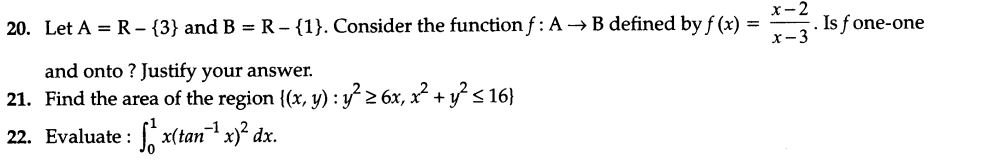# CBSE Sample Papers for Class 12 Maths Solved 2016 Set 8

0
205

SECTION —A

Question numbers 1 to 6 carry 1 mark each.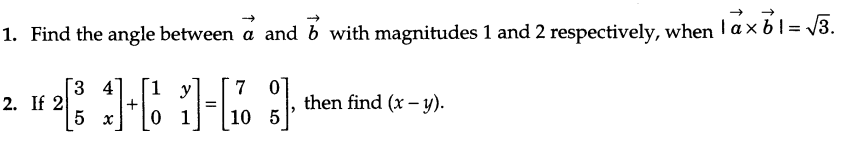3.At what points of the ellipse 16x2 + 9y2 = 400, does the coordinates decrease at the same rate at which the abscissa increases.
4.Write the direction cosines of the line joining the points (1,0,0) and (0,1,1).
5.Find the differential equation y = ex + 1.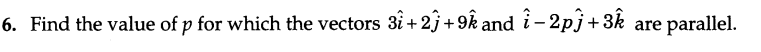SECTION — B

Question numbers 7 to 19 carry 4 mark each.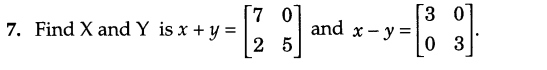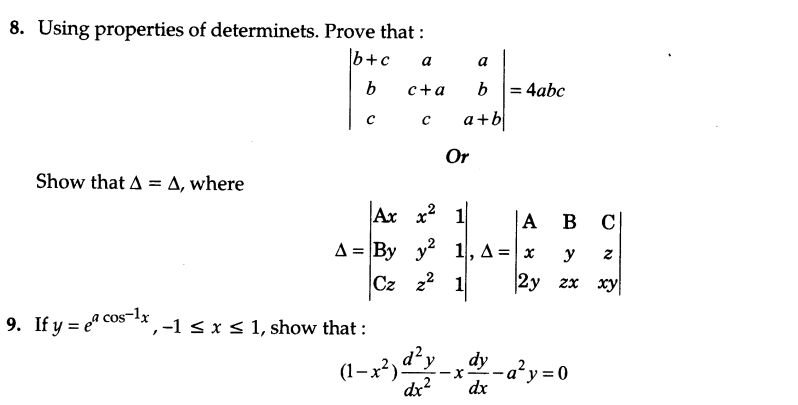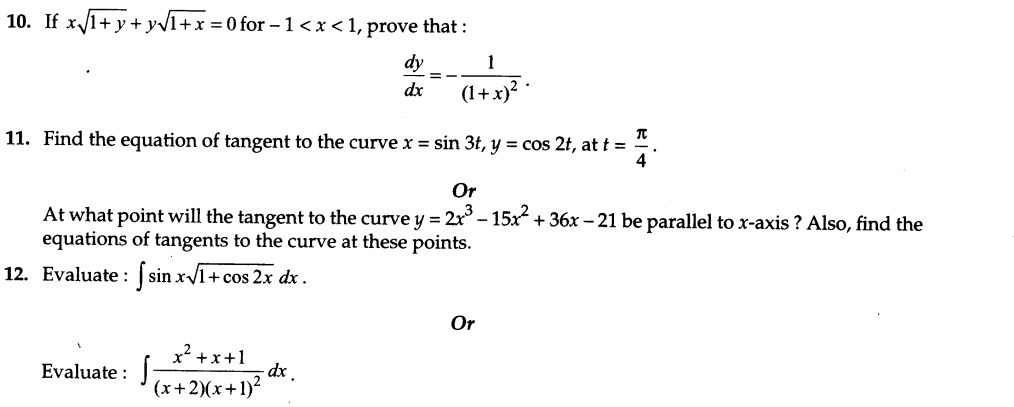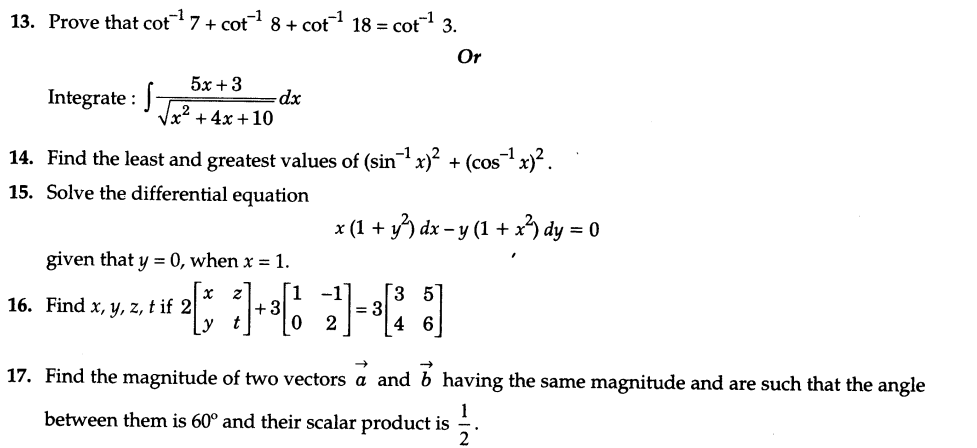18.For 6 trials of an experiment, let x be a binomial variate which satisfies the relation 9p(x = 4) = p(x = 2). Find the probability of success.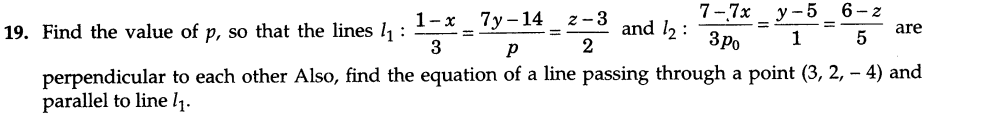SECTION — C

Question numbers 20 to 26 carry 6 mark each.